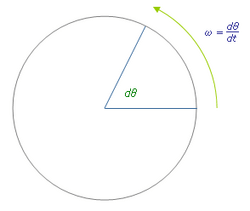# Angular velocity facts for kids

Kids Encyclopedia FactsAngular velocity describes the speed of rotation and the orientation of the axis about which the rotation takes place. The direction of the angular velocity vector will be along the axis of rotation; in this case (counter-clockwise rotation) the vector points toward the viewer.

In physics, the angular velocity specifies the angular speed at which an object is rotating along with the direction in which it is rotating.

It is a vector quantity. The SI unit of angular velocity is radians per second. But it may be measured in other units as well (such as degrees per second, degrees per hour, etc.). When it is measured in cycles or rotations per unit time (e.g. revolutions per minute), it is often called the rotational velocity and its magnitude the rotational speed. Angular velocity is usually represented by the symbol omega (Ω or ω). The direction of the angular velocity vector is perpendicular to the plane of rotation, in a direction which is usually specified by the right hand rule.

## Related pagesAngular velocity Facts for Kids. Kiddle Encyclopedia.